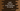# 2 ways to find the first and last character of a string in Swift## How to find the first and last character of a string in Swift:

We can get the first and last character of a string in `Swift` in different ways. In this post, I will show you two different ways to read the first and last characters of a given string.

### Method 1: By using the `first` and `last` properties of `String`:

The `first` and `last` properties of a `String` are defined as:

``````var first: Character?
var last: Character?``````

Both are optional values and return the first and the last characters of a `String`. For an empty string, the properties will be `nil`. By using these two properties, we can get the first and the last characters of a `String` as shown in the following example:

``````let givenString = "hello world"

if let firstChar = givenString.first{
print(firstChar)
}

if let lastChar = givenString.last{
print(lastChar)
}``````

Get the code on GitHub

It will print:

``````h
d``````

### Method 2: By using the index of first and last characters:

We can use the below two properties to get the first and last index of a string:

``````var startIndex: String.Index

var endIndex: String.Index``````

The `endIndex` variable is the index after the last index. So, we need to use the following method to get the index just before it or the last index of the string:

``func index(before: String.Index) -> String.Index``

With these values, we can get the first and last character of a `String`:

``````let givenString = "hello world"

print(givenString[givenString.startIndex])
print(givenString[givenString.index(before: givenString.endIndex)])``````

Get the code on GitHub